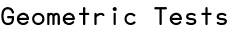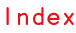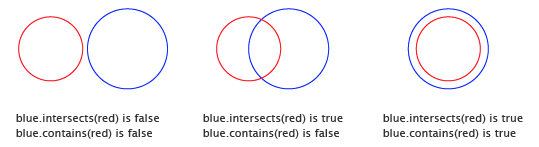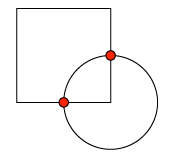RSS

Scriptographer offers various functionalities for testing the overlapping of paths and finding their intersections.To find out if one path intersects another, we can use path.intersects(path):

```// Create a circular path at {x: 100, y: 100}, with a radius of 50:
var blue = new Path.Circle([100, 100], 50);
blue.strokeColor = 'blue';

// Create a second circular path that overlaps the first path:
var red = new Path.Circle([50, 100], 50);
red.strokeColor = 'red';

console.log(red.intersects(blue)); // true
```

We can find out if an item is contained within the interior of a path by using path.contains(item):

```// Create a circular path at {x: 100, y: 100}, with a radius of 50:
var blue = new Path.Circle([100, 100], 50);
blue.strokeColor = 'blue';

// Create a second circular path that overlaps the first path,
// but isn't contained within its interior:
var red = new Path.Circle([50, 100], 40);
red.strokeColor = 'red';

// This will return false, because the red path isn't completely
// within the interior of the blue path:
console.log(blue.contains(red)); // false

// Now move the red path to the same position as the blue path:
red.position = blue.position;

// Now the contains function returns true, because secondPath is
// completely within the interior of firstPath:
console.log(blue.contains(red)); // true
```

## Finding Path Intersections

Scriptographer allows you to find the intersections between paths by using the path.getIntersections(path) function. When intersections are found, the function returns an array of CurveLocation objects, which describe the intersection locations on the intersected Curve of the path.

A CurveLocation object contains different kinds of information about the intersection, including:

## Path Intersections Example

The following example script creates two overlapping circle shaped paths and draws red circles on the positions of their intersections.```// Create two overlapping paths:
var square = new Path.Rectangle(new Point(0, 0), new Size(50, 50));
var circle = new Path.Circle(square.bounds.bottomRight, 25);

// Find the intersection locations between the paths:
var locations = square.getIntersections(circle);

// Loop through the locations (if any):
for(var i = 0; i < locations.length; i++) {

// The position of the intersection:
var point = locations[i].point;

// Create a circle shaped path at the
// position of the intersection:
var path = new Path.Circle(point, 2);
path.fillColor = 'red';
}
```

## Mouse Tool Example

The following example shows an example of a mouse tool that creates a circle shaped path every time you click and shows the intersections with the last path while you drag the mouse.

```// Define these variables outside of the mouseHandlers, so
// we can access them from both mouse handlers:
var path, lastPath;

function onMouseDown(event) {
// if a path was produced before, put it into the
// lastPath variable, which we will be checking for
// intersections later.
if(path) {
lastPath = path;
}
path = new Path.Circle(event.point, 50);
}

function onMouseDrag(event) {
// Move path to the position of the mouse:
path.position = event.point;

// If we already have a lastPath:
if(lastPath) {
// Find the intersections between path and lastPath, if any.
var intersections = path.getIntersections(lastPath);

// Check to see if there were any intersections:
if(intersections.length) {
// Run through the intersections:
for(var i = 0, i < intersections.length; i++) {
// The point of the intersection:
var point = intersections[i].point;

// Create a red circle shaped path with its
// centerpoint on the intersection point:
var hitPath = new Path.Circle(point, 5);
hitPath.fillColor = 'red';

// Automatically remove the path, the next time
// onMouseDrag is called:
hitPath.removeOn({ drag: true });
}
}
}
}
```